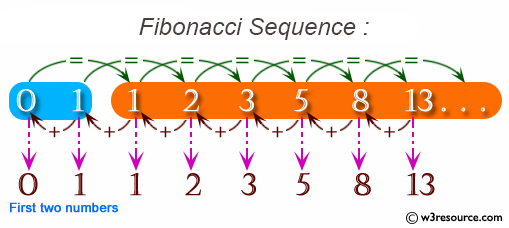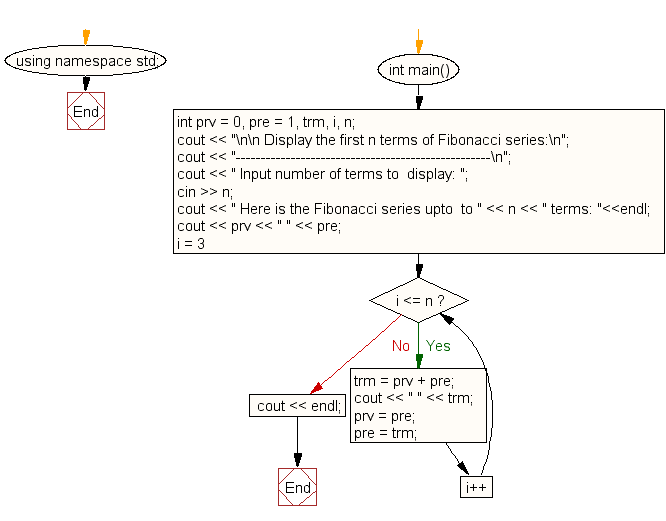﻿ C++ Exercises: Display the first n terms of Fibonacci series - w3resource

# C++ Exercises: Display the first n terms of Fibonacci series

## C++ For Loop: Exercise-27 with Solution

Write a program in C++ to display the first n terms of the Fibonacci series.

Pictorial Presentation:Sample Solution:-

C++ Code :

``````#include <iostream>
using namespace std;

int main()
{
int prv = 0, pre = 1, trm, i, n;
cout << "\n\n Display the first n terms of Fibonacci series:\n";
cout << "---------------------------------------------------\n";
cout << " Input number of terms to  display: ";
cin >> n;
cout << " Here is the Fibonacci series upto  to " << n << " terms: "<<endl;
cout << prv << " " << pre;
for (i = 3; i <= n; i++)
{
trm = prv + pre;
cout << " " << trm;
prv = pre;
pre = trm;
}
cout << endl;
}
``````

Sample Output:

``` Display the first n terms of Fibonacci series:
---------------------------------------------------
Input number of terms to  display: 10
Here is the Fibonacci series upto  to 10 terms:
0 1 1 2 3 5 8 13 21 34
```

Flowchart:C++ Code Editor: